### Home > PC > Chapter 2 > Lesson 2.3.8 > Problem2-174

2-174.
1. Write each equation in exponential form. Homework Help ✎

1. log4 16 = 2

2. log6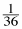= −2

3. log 1000 = 3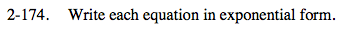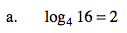A log is an exponent. In this case, the exponent equals 2.

The 4 is the base.

16 is the answer to the exponential question.

42 = 16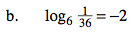Use the same thinking process as (a) above.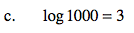This is called a 'common log'. The base is 10.